## Main

The geometry and topology in Hilbert space constitute a central issue in quantum physics, which has recently also shed a new light on the electronic states in solids. The wavefunctions are characterized by the Berry connection between two neighbouring points, in both momentum space and real space, which plays the role of the vector potential leading to the concept of emergent electromagnetic field (EEMF). Global topology of the manifold in Hilbert space is represented by topological integers. As integers cannot change continuously, it gives a certain stability to the system. For example, the Chern number, which is given by the integral of the emergent magnetic field over the first Brillouin zone, protects the surface or edge states supporting the dissipationless current flows (that is, bulk–edge correspondence). The one-dimensional chiral edge mode in the quantum Hall effect and the Dirac surface state in three-dimensional topological insulators (TI; refs 7,8) are the representative examples of this phenomenon. In addition, it has recently been proposed9,10 and observed11,12,13,14 that TI with doped magnetic ions—namely, Cr or V—produces the quantized version of the anomalous Hall effect (AHE) in the absence of an external magnetic field.

Topology in real space, on the other hand, is exemplified by skyrmion spin texture15,16,17 found in chiral-lattice magnets such as MnSi (ref. 15) or Fe1−xCoxSi (ref. 16). Here, the solid angle subtended by the spins forms an emergent magnetic field in real space, and its integral over the two-dimensional space defines a topological integer called the skyrmion number. Namely, the skyrmion number counts the number of times the spin direction wraps around a unit sphere. This integer protects the skyrmion from annihilation and allows it to behave as a single particle.

Thus far, the EEMF in momentum and real spaces have mostly been studied separately. Recent advances in fabricating artificial structures of materials, however, enable the design of emergent phenomena considering both momentum and real spaces in a unified way. Here, we examined these geometric Hall effects in TI heterostructures composed of magnetic TI Crx(Bi1−ySby)2−xTe3 (CBST) and non-magnetic TI (Bi1−ySby)2Te3 (BST; refs 11,12,13,18,19,20,21,22), which were grown on InP(111) substrates using molecular-beam epitaxy20,21,22. By applying a field-effect transistor structure (FET, see Methods), the Fermi energy (EF) of the TI heterostructures can be precisely controlled in a large energy range over the bulk bandgap. In particular, the quantum AHE realized in the magnetic TIs offers an interesting scenario where the two different physical mechanisms merge—namely, skyrmion formation around the doped carrier into the quantum Hall ferromagnet23 and skyrmion formation at the interface of magnets24,25,26,27. First, we investigate the gate voltage (VG) and temperature (T) dependence of the Hall effect for a 2-nm CBST/5-nm BST heterostructure as shown in Fig. 1a. With increasing VG at 2 K (Fig. 1b), the hysteresis curves of the Hall conductivity σxy gradually change shape, demonstrating a maximum value at VG = 0.2 V . In Fig. 1c, d, we plot the temperature dependence at selected voltages VG = 0.2 V and −7.0 V. In contrast to the conventional temperature dependence of magnetic TI shown in Fig. 1c at VG = 0.2 V, where EF is close to the Dirac point, we find two notable features under hole accumulation at VG = −7.0 V in Fig. 1d: first, sign-reversal of the anomalous Hall conductivity σAxy (Hall conductivity under zero magnetic field) at 6 K and, second, non-monotonous behaviour of σxy as a function of magnetic field. In this paper, we first study the former feature and discuss the latter afterwards, both from the viewpoint of the geometric Hall effect. The detailed data set of σAxy in TVG parameter space (Fig. 1e) shows the sign-reversal roughly at negative VG and in the 6–20 K region. Such a sign-reversal of σAxy has not been observed in single-layer CBST, which always gives a positive sign regardless of its EF position and temperature11,12,13, as exemplified in Fig. 2a. Hence, such a transition between positive and negative σAxy is a distinct behaviour in the 2-nm/5-nm (Fig. 1d) and 3-nm/5-nm (Fig. 2b) heterostructures. Note that the longitudinal conductivity (approximately 102 (Ω cm)−1) is so low that each anomalous component cannot be of an extrinsic origin1, such as skew scattering; therefore, an intrinsic origin should be explored in the electronic band structure characteristic of the TI heterostructures.Figure 1: Anomalous Hall conductivity of a topological insulator (TI) heterostructure.

To address this issue, we theoretically study the low-energy effective Hamiltonian of the TI heterostructures (see Methods). We consider two cases, a 10-nm CBST single layer and a 5-nm CBST/5-nm BST heterostructure, as shown in Fig. 3a, d. The band structure obtained for CBST (Fig. 3b) shows gapped Dirac surface bands in addition to the bulk bands. In the case of CBST/BST (Fig. 3e), the Dirac band at the top surface of the CBST is gapped owing to the exchange coupling, whereas the Dirac band at the bottom surface of the BST remains gapless. Moreover, the top of the valence bands shows Rashba splitting because of the broken spatial inversion symmetry in the heterostructures (red lines in the lower inset of Fig. 3e), and these Rashba split bands are slightly gapped owing to the exchange coupling (black lines). In fact, such Rashba splitting in the bulk band of TI has also been observed in previous angle-resolved photoemission spectroscopy (ARPES) studies28. In Fig. 3c, f, we show the Hall conductivity obtained from the Berry curvature that encodes the nontrivial geometry in the momentum space. In both cases, σxy is maximized when EF is within the gap of the surface bands. In addition, σxy in Fig. 3f shows a sharp negative peak at the top of the valence bands, denoted by a red triangle. This is attributed to the slightly gapped Rashba split bands on which the Berry curvature is concentrated. Moreover, the negative peak decreases for larger exchange coupling, which corresponds to the case of lower temperature (see Supplementary Information for the details). This means that a positive AHE component is dominant at lower temperatures. These theoretical arguments coincide well with the experimental results shown in Fig. 1e, g, in which the band energy scheme is based on the results of σxy at zero and high (14 T) fields, including ν = 1 and ν = 0 quantum Hall plateaux (Fig. 1f; ref. 20).

In addition to the sign-reversal, we observe anomalous magnetic-field-dependent behaviours in the Hall effect in 2-nm CBST/5-nm BST heterostructures, as shown in Figs 2c and 1d. To elucidate the hysteretic anomaly in the Ryx versus B curve we compared Ryx′ (= RyxR0B) with the magnetization of the film (see Methods) in the top panel of Fig. 2d. Here, R0 is the ordinary Hall coefficient determined from the linear slope at high magnetic field (see Supplementary Information). In the conventional theory, Ryx′ is nothing other than the anomalous Hall term, which is proportional to the magnetization M. In reality, however, a large discrepancy between Ryx′ and M is discerned; we need to add the nontrivial Hall component (shown in the bottom panel of Fig. 2d) as well as the M-linear anomalous Hall term to reproduce the observed Ryx′. As a plausible origin, we propose skyrmion formation from both theoretical and experimental aspects. Under skyrmion spin texture, the moving electrons experience the EEMF, giving rise to an additional Hall component termed the topological Hall effect (THE, note that ‘topological’ is defined in real space)3,4, which is observed in the skyrmion phase of some chiral-lattice magnets5,6 as well as in frustrated magnets endowed with scalar spin chirality29,30. The magnetic-field dependence of THE shows a hysteresis behaviour; the magnetic field showing the maximal THE is observed to shift slightly to lower field as a whole by up to 0.02 T with decreasing sweep rate from 2 × 10−3 T s−1 to 3 × 10−5 T s−1 (see Supplementary Information for the details).

To confirm the possibility of skyrmion formation, we simulated the energetic stability of a skyrmion using a three-dimensional tight-binding model (see Methods). We consider the following three cases: Bloch (Fig. 4a), Néel1 (Fig. 4b) and Néel2 (Fig. 4c) for a CBST single layer (Fig. 4d) and a CBST/BST heterostructure (Fig. 4h). In Fig. 4e–g, i–k, we show the formation energy of a skyrmion relative to that of the spin-collinear ferromagnet as a function of the skyrmion radius R at various doping levels. For the CBST single layer in Fig. 4e–g, the energy is minimized always at R = 0, implying that the ferromagnetic state is the ground state regardless of EF. For the electron-doped (Fig. 4i) or half-filling (Fig. 4j) cases of the CBST/BST heterostructure, the situation is similar. In the hole-doped case in the heterostructure (Fig. 4k), by contrast, a negative energy region exists for the Néel2-type skyrmion (R ≠ 0), denoted by a red triangle. The emergence of a stable condition for skyrmion formation can be explained as follows. The surface state of the TI exhibits inversion symmetry breaking and a fairly strong spin–orbit interaction, as exemplified by the spin–momentum-locking in the Dirac dispersion. Thus, the electrons at the surface state mediate the Dzyaloshinskii–Moriya (DM) interaction where the DM vector is pointing in the in-plane direction24,25,26,27,31. In the heterostructure, therefore, such an in-plane DM vector originating from the top CBST surface favours the formation of a Néel-type skyrmion24,25,26,27. However, in the single-layer CBST, DM vectors exist both at the top and bottom surface, each pointing along opposite directions, so that skyrmion formation is disfavoured owing to the frustration. Hence, broken spatial inversion symmetry associated with the heterostructure is the essential requirement for skyrmion formation.

Theoretical verification of skyrmion formation at the hole-accumulated condition agrees well with the experimental situation at large negative VG (−7.0 V), as shown in Fig. 1d. In 2-nm/5-nm heterostructures (Fig. 2c), the topological Hall components RTyx are identified as the green region, which is shown in Fig. 2e in the BT parameter space. From this plot, we can see that skyrmions are formed in the course of magnetization reversal in a wide temperature range below the Curie temperature. The maximum THE, amounting to 140 Ω (lower panel of Fig. 2d), corresponds to the magnitude of an emergent magnetic flux density of 0.29 T, taking into consideration the normal Hall coefficient of 490 Ω T−1 (see Supplementary Information). However, in the 3-nm CBST/5-nm BST heterostructure, no THE is observed, although sign-reversal of AHE is discerned, as shown in Fig. 2b. (As for the reproducibility of such a critical CBST-thickness-dependent THE, see Supplementary Information.) This observation implies the importance of the DM interaction; as the CBST thickness increases, the relative strength of the exchange interaction to the DM interaction increases, such that skyrmion formation would become less favoured. From this viewpoint, further optimization of the magnetic/non-magnetic TI heterostructure may open a way to control skyrmions on TI.

## Methods

### MBE thin film growth.

Thin films were grown by molecular-beam epitaxy (MBE) on insulating InP (111) substrates. The growth temperatures for BST and CBST are 220 °C and 200 °C, respectively. The Cr (x 0.2) content is estimated from the beam equivalent pressure (BEP) ratio through Cr/(Bi + Sb). The Te flux (BEP = 1.0 × 10−4 Pa) was over-supplied while keeping the Te/(Bi + Sb) ratio close to 20 to suppress Te vacancies. Under this condition, the growth rate is about 0.2 nm min−1. Before the growth of the first layer, a monolayer Sb2Te3 buffer layer was grown to give a better morphology and epitaxy. After epitaxial growth of the BST layer, annealing under exposure to the Te flux was performed in situ at 380 °C to get a smoother surface. The same procedure was employed for the following CBST layer. To suppress degradation of the film, an AlOx capping layer with a thickness of approximately 3 nm was deposited at room temperature by an atomic layer deposition system immediately after removing the samples from the MBE vacuum chamber. Please note that Cr interdiffusion in the heterostructure is negligible, as confirmed by energy-dispersive X-ray spectroscopy imaging (see Supplementary Information). A list of sample structures, such as film thickness, and Bare or FET (including applied gate voltages), is summarized in Supplementary Note 1 and Supplementary Table 1.

### FET device fabrication.

The Hall-bar device pattern was defined by a photolithographic technique and Ar ion-milling processes. After defining the Hall-bar structure, AlOx with a thickness of approximately 20 nm was deposited to serve as a gate capacitor. For Ohmic-contact electrodes and a top gate electrode, 5-nm Ti/45-nm Au were deposited by means of an electron-beam evaporator.

### Transport and magnetization measurements.

Transport measurements for the bare films were conducted using the d.c. transport option of a physical property measurement system (PPMS, Quantum Design). FET devices were measured in the PPMS with a standard lock-in technique at low frequency (7 Hz) and with a low excitation current (10 nA) to suppress heating effects. Low-temperature (<2 K) measurements were performed using the 3He option of the PPMS. Magnetization measurements for the bare film of 2-nm CBST/5-nm BST were conducted using a magnetic property measurement system (MPMS, Quantum Design). All the transport and magnetization data were anti-symmetrized as a function of the magnetic field.

### Calculation of band structures and anomalous Hall conductivity.

Band structures and anomalous Hall conductivity were calculated using the low-energy effective Hamiltonian of the TI heterostructures given by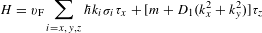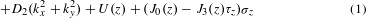Here, σi and τi are the Pauli matrices for the spin and orbital degrees of freedom. τz = + 1 for Bi/Sb p-orbitals and τz = −1 for Te p-orbitals. The x and y directions are parallel to the TI thin films and periodic, whereas the z direction is perpendicular to the TI thin films and not periodic. Therefore, kz should be regarded as the operator −i∂z. We adopted the following parameters in numerical calculations: vF = 5.0 × 105 m s−1, m = −300 meV, D1 = 60 eV Å2, D2 = 20 eV Å2 inside the TI thin films8. The asymmetry between the conduction and valence bands is captured by the D2 and J3 term. We introduced the potential asymmetry between BST and CBST by setting U(z) = 0 meV for BST and U(z) = −40 meV for CBST, and the exchange couplings in magnetic TI by setting J0 = J3 = 0 meV for BST and J0 = 5 meV and J3 = 1 meV for CBST. Here, the parameters J0 and J3 are symmetric and asymmetric parts of the exchange couplings for the two orbitals31. The exchange coupling for Te p-orbitals should be slightly larger than that for Bi/Sb p-orbitals because Te atoms locate nearer to doped Cr ions. (This asymmetry is captured by setting J3 = 0.2J0.) The anomalous Hall conductivity is obtained by integrating the Berry curvature Bk = × Ak over the momentum space, where Ak is the Berry connection Ak = −iψk | k | ψk〉 with Bloch wavefunctions ψk. As seen in Fig. 3e, the valence top bands show Rashba splitting due to the broken inversion symmetry in the TI heterostructure. This Rashba splitting results in the concentration of the Berry curvature at the valence top bands and a large contribution to the anomalous Hall conductivity there.

### Calculation of skyrmion stability.

The stability of a skyrmion was calculated by the three-dimensional tight-binding model for TI, corresponding to the effective Hamiltonian in the continuum approximation given in equation (1),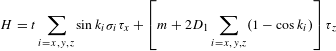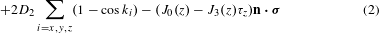where σi and τi are the Pauli matrices for the spin and the orbital degrees of freedom. The system size is Lx = Ly = 31 sites, Lz = 8 layers, and the periodic boundary condition for the x and y directions is imposed. In this model, the D2 and J3 terms break the particle–hole symmetry31 as mentioned above, and they lead to asymmetry between the electron-doped and hole-doped cases. We have set D1 = 1, D2 = 0.2, t = 1, m = −1, which realizes the strong TI phase. The exchange couplings in magnetic TI are set as J0 = J3 = 0 for BST and J0 = 0.1 and J3 = 0.02 for CBST. This choice of the parameters is determined to reduce the finite-size effect, and hence the numerical results are not regarded as quantitatively corresponding to the real system, but rather as qualitative information.

The skyrmion configuration n(r) is set as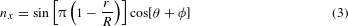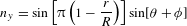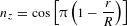for r < R, and nz = 1 for the outer region r < R. The skyrmion radius R is set as the variational parameter and φ determines the magnetic helicity or the skyrmion type—that is, the Bloch type for φ = (π/2), the Néel1 type for φ = 0 and the Néel2 type for φ = π. We note that R = 0 corresponds to the ferromagnetic state. One can interpret the obtained results presented in the main text from the facts that valence bands are more strongly coupled to the magnetic ions as a result of the J3 term, and the DM interaction is induced from the spin–momentum-locking in the surface state of TI.